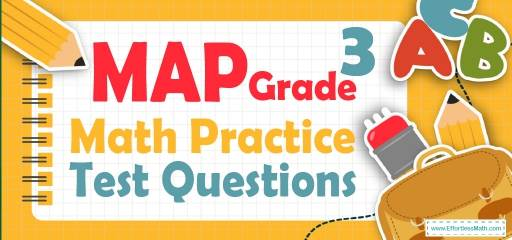# 3rd Grade MAP Math Practice Test QuestionsPreparing your student for the 3rd Grade MAP Math test? Help your students build MAP Math test skills by following common 3rd Grade MAP Math questions. Practicing common math questions is the best way to help your students improve their Math skills and prepare for the test. Here, we provide a step-by-step guide to solve 10 common MAP Math practice problems covering the most important math concepts on the 3rd Grade MAP Math test.

## 10 Sample 3rd Grade MAP Math Practice Questions

1- Which number correctly completes the subtraction sentence
$$8000 – 658 =$$ ___________ ?

A. 7342

B. 7452

C. 742

D. 7458

2- Jason packs 14 boxes with flashcards. Each box holds 40 flashcards. How many flashcards Jason can pack into these boxes?

A. 56

B. 480

C. 540

D. 560

3- Which of the following statements describes the number 24,589?

A. The sum of two thousands, 4 thousands, five hundreds, eighty tens, and nine ones

B. The sum of forty thousands, 2 thousands, five hundreds, eight tens, and nine ones

C. The sum of twenty thousands, 4 thousands, fifty hundreds, eighty tens, and nine ones

D. The sum of twenty thousands, 4 thousands, five hundreds, eight tens, and nine ones

4- What is the value of “A” in the following equation?
$$21 + A + 9 = 44$$

A. 10

B. 12

C. 14

D. 20

5- Mr. smith usually eats four meals a day. How many meals does he eat in a week?

A. 21

B. 24

C. 28

D. 30

6- What is the value of $$A$$ in the equation $$72 ÷ A = 8$$

A. 2

B. 6

C. 7

D. 9

7- Use the models below to answer the question.

Which statement about the models is true?

A. Each shows the same fraction because they are the same size.

B. Each shows a different fraction because they are different shapes.

C. Each shows the same fraction because they both have 3 sections shaded.

D. Each shows a different fraction because they both have 3 shaded sections but a different number of total sections.

8- To what number is the arrow pointing?

A. 36

B. 38

C. 30

D. 42

9- Emily has 108 stickers and she wants to give them to nine of her closest friends. If she gives them all an equal number of stickers, how many stickers will each of Emily’s friends receive? ___________

10- The following models are the same size and each divided into equal parts.
The models can be used to write two fractions.

Based on the models, which of the following statements is true?

A. $$\frac{2}{8}$$ is bigger than $$\frac{4}{16}$$.

B. $$\frac{2}{8}$$ is smaller than $$\frac{4}{16}$$.

C. $$\frac{2}{8}$$ is equal to $$\frac{4}{16}$$.

D. We cannot compare these two fractions only by using the models.

## Best 3rd Grade MAP Math Prep Resource for 2021

1- A
$$8000 – 658 = 7342$$

2- D
$$14 × 40 = 560$$

3- D
24,589 is the sum of $$20,000; 4,000; 500; 80;$$ and 9

4- C
$$A = 44 – (21 + 9)$$
$$A = 14$$

5- C
In 1 day 4 meals so $$4 × 7 = 28$$ meals a week.

6- D
$$A = 72 ÷ 8$$
$$A= 9$$

7- D
the model for the first fraction is divided into 6 equal parts. We shade 3_6 to show the same amount as 1_2. The model for the second fraction is divided into 8 equal parts. We shade 3_ 8 that it shows this two model are different fractions.

8- C
The arrow shows exactly the middle of two numbers 25 and 35, so the answer is 30

9- 12
$$108 ÷ 9 = 12$$

10- C
the model for the first fraction is divided into 8 equal parts. We shade 2_ 8 to show the same amount as 1_ 4. The model for the second fraction is divided into 16 equal parts. We shade 4_ 16 to show the same amount as 1_ 4.

### What people say about "3rd Grade MAP Math Practice Test Questions - Effortless Math: We Help Students Learn to LOVE Mathematics"?

No one replied yet.

X
24% OFF

Limited time only!

Save Over 24%

SAVE $5 It was$20.99 now it is \$15.99